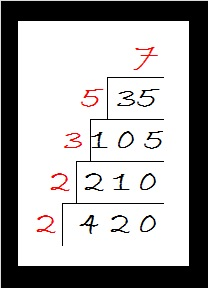# 1125 May I Interest You In a Little Cake?

1 + 1 + 2 + 5 = 9, so 1125 can be evenly divided by both 3 and 9.
1125 can also be evenly divided by 5 because the last digit can be divided by 5.
1125 can be evenly divided by 25 because the last two digits can be divided by 25.
1125 can be evenly divided by 125 because the last three digits can be divided by 125.

May I interest you in a little cake? Although I’ve put many factor trees on this website, I actually prefer making factor cakes. I like them because all the prime factors can easily be found IN ORDER on the outside of the cake. The more layers the factor cake has, the more I like it. Here is THE factor cake for 1125: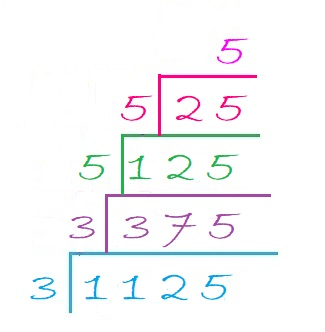As you can see, the prime factorization of 1125 is 3 × 3 × 5 × 5 × 5. All of its prime factors are written nicely in order. None of them are hiding anyplace like they might in a factor tree.

Here are some facts about the number 1125:

• 1125 is a composite number.
• Prime factorization: 1125 = 3 × 3 × 5 × 5 × 5, which can be written 1125 = 3² × 5³
• The exponents in the prime factorization are 2 and 3. Adding one to each and multiplying we get (2 + 1)(3 + 1) = 3 × 4 = 12. Therefore 1125 has exactly 12 factors.
• Factors of 1125: 1, 3, 5, 9, 15, 25, 45, 75, 125, 225, 375, 1125
• Factor pairs: 1125 = 1 × 1125, 3 × 375, 5 × 225, 9 × 125, 15 × 75, or 25 × 45
• Taking the factor pair with the largest square number factor, we get √1125 = (√225)(√5) = 15√5 ≈ 33.541021125 is the hypotenuse of three Pythagorean triples:
675-900-1125 which is (3-4-5) times 225,
315-1080-1125 which is (7-24-25) times 45, and
396-1053-1125 which is 9 times (44-117-125)

1125 looks interesting to me when it is written in these bases:
It’s 5A5 in BASE 14 (A is 10 base 10) because 5(14²) + 10(14) + 5(1) = 1125
500 in BASE 15 because 5(15²) = 1125, and
it’s 3F3 in BASE 17 (F is 15 base 10) because 3(17²) + 15(17) + 3(1) = 1125

# 1122 Factor Trees

We can easily tell that 1122 is divisible by 2 because it is even.
It is divisible by 3 because 1 + 2 = 3, no matter how many times you do it.
11 and 22 are both divisible by 11, so obviously, 1122 is as well.
1122 is also divisible by 17, but there isn’t an easy trick to use to find that out without just dividing it.

There are 24 ways to arrange the four factors of 1122. Each of those 24 ways can be the bottom four numbers of a factor tree (See the first three trees below.) or the numbers running down the side of a factor tree (See the last four trees below.).1122 makes some very well proportioned factor trees!

• 1122 is a composite number.
• Prime factorization: 1122 = 2 × 3 × 11 × 17
• The exponents in the prime factorization are 1, 1, 1, and 1. Adding one to each and multiplying we get (1 + 1)(1 + 1)(1 + 1)(1 + 1) = 2 × 2 × 2 × 2 = 16. Therefore 1122 has exactly 16 factors.
• Factors of 1122: 1, 2, 3, 6, 11, 17, 22, 33, 34, 51, 66, 102, 187, 374, 561, 1122
• Factor pairs: 1122 = 1 × 1122, 2 × 561, 3 × 374, 6 × 187, 11 × 102, 17 × 66, 22 × 51, or 33 × 34
• 1122 has no square factors that allow its square root to be simplified. √1122 ≈ 33.496271122 is the product of two consecutive number: 33 × 34 = 1122
That makes 1122 the sum of the first 33 even numbers:
2 + 4 + 6 + 8 + . . . + 62 + 64 + 66 = 1122

1122 is the hypotenuse of a Pythagorean triple:
528-990-1122 which is (8-15-17) times 66

1122 is palindrome 2G2 in BASE 20 (G is 16 base 10)
because 2(20²) + 16(20) + 2(1) = 1122

# 1120 There’s Lots of Shade under These Factor Trees!

It’s hot this summer, but you can rest under the shade of some of 1120’s factor trees. Whether you want a tall tree or a wide one, these are only a few of the MANY possible ones you can choose. Every one of them gives you the same prime factors which are shown in pink here.Here are some things I’ve learned about the number 1120:

• 1120 is a composite number.
• Prime factorization: 1120 = 2 × 2 × 2 × 2 × 2 × 5 × 7, which can be written 1120 = 2⁵ × 5 × 7
• The exponents in the prime factorization are 5, 1, and 1. Adding one to each and multiplying we get (5 + 1)(1 + 1)(1 + 1) = 7 × 2 × 2 = 28. Therefore 1120 has exactly 28 factors.
• Factors of 1120: 1, 2, 4, 5, 7, 8, 10, 14, 16, 20, 28, 32, 35, 40, 56, 70, 80, 112, 140, 160, 224, 280, 560, 1120
• Factor pairs: 1120 = 1 × 1120, 2 × 560, 4 × 280, 5 × 224, 7 × 160, 8 × 140, 10 × 112, 14 × 80, 16 × 70, 20 × 56, 28 × 40, or 32 × 35
• Taking the factor pair with the largest square number factor, we get √1120 = (√16)(√70) = 4√70 ≈ 33.46641120 is the sum of the twenty prime numbers from 17 to 101.
It is also the sum of these two consecutive primes:
557 + 563 = 1120

1120 is the hypotenuse of exactly one Pythagorean triple:
672-896-1120 which is (3-4-5) times 224

I like the way 1120 looks in these other bases:
It’s 1112111 in BASE 3 because 3⁶ + 3⁵ + 3⁴ + 2(3³) + 3² + 3  + 1 = 1120,
929 in BASE 11 because 9(11²) + 2(11) + 9(1) = 1120,
WW in BASE 34 (W is 32 base 10) because 32(34) + 32(1) = 32(35) = 1120,
and W0 in BASE 35 because 32(35) = 1120

# 1092 Predictable Factor Trees

A couple of years ago on Memorial Day weekend, we bought two peach trees from a local nursery. Those trees have grown bigger, and we will get some peaches this year. The smaller tree has lots of fruit growing on it and will need some attention because the branches will be too small to support the weight of all that fruit. The bigger tree has exactly one peach growing on it.

These factor trees for the number 1092 are a lot more predictable than those peach trees: No matter which of its factor pairs you use, you will always eventually get
2² × 3 × 7 × 13 = 1092.Here are some more facts about 1092:

• 1092 is a composite number.
• Prime factorization: 1092 = 2 × 2 × 3 × 7 × 13, which can be written 1092 = 2² × 3 × 7 × 13
• The exponents in the prime factorization are 2, 1, 1, and 1. Adding one to each and multiplying we get (2 + 1)(1 + 1)(1 + 1)(1 + 1) = 2 × 3 × 2 × 2 = 24. Therefore 1092 has exactly 24 factors.
• Factors of 1092: 1, 2, 3, 4, 6, 7, 12, 13, 14, 21, 26, 28, 39, 42, 52, 78, 84, 91, 156, 182, 273, 364, 546, 1092
• Factor pairs: 1092 = 1 × 1092, 2 × 546, 3 × 364, 4 × 273, 6 × 182, 7 × 156, 12 × 91, 13 × 84, 14 × 78, 21 × 52, 26 × 42, or 28 × 39
• Taking the factor pair with the largest square number factor, we get √1092 = (√4)(√273) = 2√273 ≈ 33.04542(12 × 13 × 14)/2 = 1092
Even though 1092 can claim that cool fact, it will actually make the next number a STAR!

1092 is the sum of the twelve prime numbers from 67 to 113:
67 + 71 + 73 + 79 + 83 + 89 + 97 + 101 + 103 + 107 + 109 + 113 = 1092

1092 has a lot of factors, but it is the hypotenuse of only one Pythagorean triple:
420-1008-1092 which is (5-12-13) times 84

Look how cool 1092 is in some other bases:

Not only does it use only 0’s and 1’s in base 2 but also in consecutive bases 3 and 4:
It’s 10001000100 in BASE 2 because 2¹º + 2⁶ + 2² = 1092,
1111110 in BASE 3 because 3⁶ + 3⁵ + 3⁴ + 3³ + 3² + 3¹ = 1092,
and 101010 in BASE 4 because  4⁵ + 4³ + 4¹ = 1092

I like the way it looks in consecutive bases 12 and 13:
It’s 770 in BASE 12, because 7(12² + 12) = 7(156) = 1092
and 660 in BASE 13 because 6(13² + 13) = 6(182) = 1092

And its repdigit 444 in BASE 16 because 4(16² + 16 + 1) = 1092

Some of these facts about 1092 were predictable and some were not, but I have enjoyed learning all of them and hope that you have too.

# 1050 Factor Trees Are In Bloom This Spring

1050 has much more than the average number of factors for a number its size. I decided to make a few of its MANY possible factor trees in some springtime colors. I hope you enjoy looking at them. Its prime factors, 2, 3, 5, 5, and 7, are in green.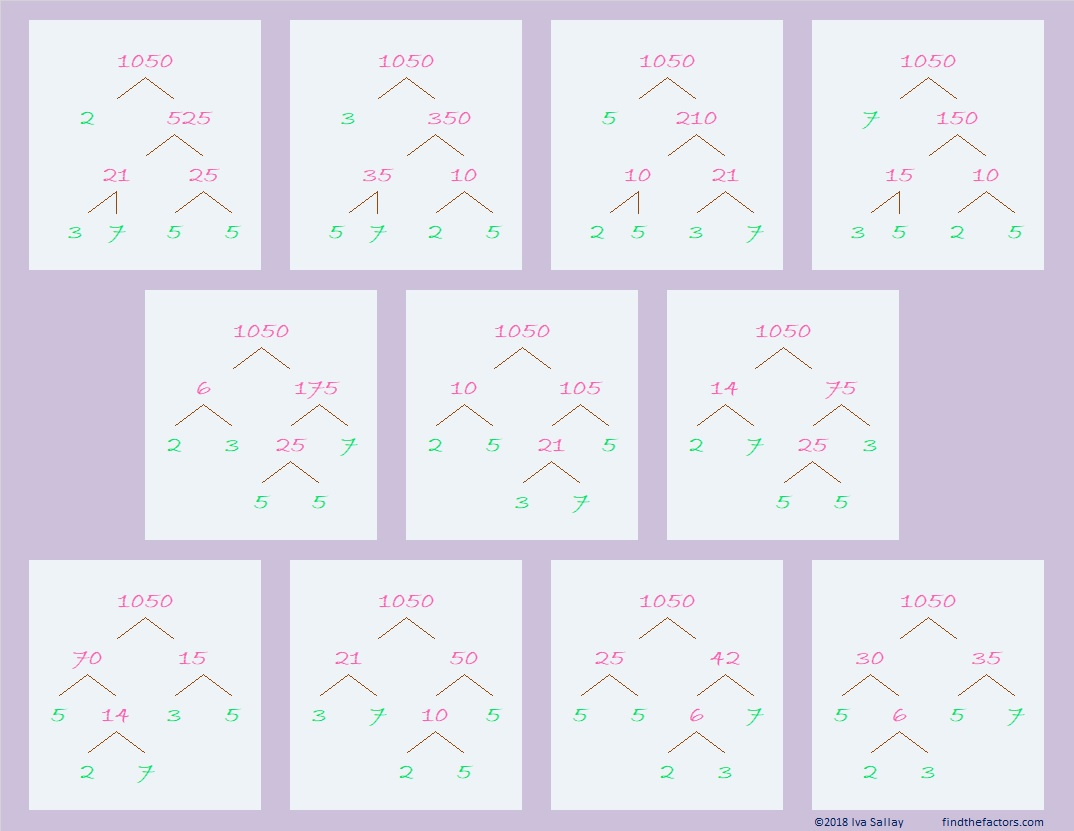What can I tell you about the number 1050?

• 1050 is a composite number.
• Prime factorization: 1050 = 2 × 3 × 5 × 5 × 7, which can be written 1050 = 2 × 3 × 5² × 7
• The exponents in the prime factorization are 1, 1, 2, and 1. Adding one to each and multiplying we get (1 + 1)(1 + 1)(2 + 1)(1 + 1) = 2 × 2 × 3 × 2 = 24. Therefore 1050 has exactly 24 factors.
• Factors of 1050: 1, 2, 3, 5, 6, 7, 10, 14, 15, 21, 25, 30, 35, 42, 50, 70, 75, 105, 150, 175, 210, 350, 525, 1050
• Factor pairs: 1050 = 1 × 1050, 2 × 525, 3 × 350, 5 × 210, 6 × 175, 7 × 150, 10 × 105, 14 × 75, 15 × 70, 21 × 50, 25 × 42, or 30 × 35
• Taking the factor pair with the largest square number factor, we get √1050 = (√25)(√42) = 5√42 ≈ 32.403703If you had \$10.50 in quarters, you would have 42 quarters because 42(25) = 1050
If you had \$10.50 in dimes, you would have 105 dimes because 105(10) = 1050
If you had \$10.50 in nickles, you would have 210 nickles because 210(5) = 1050

1050 is the sum of the twenty-two primes from 7 to 97.

1050 is the hypotenuse of two Pythagorean triples:
630-840-1050 which is (3-4-5) times 210
294-1008-1050 which is (7-24-25) times 42

1050 looks interesting to me when it is written in a couple of other bases:
It’s UU in BASE 34 (U is 30 base 10) because 30(34) + 30(1) = 30(35) = 1050,
and it’s U0 in BASE 35 because 30(35) = 1050

# A Forest of 1008 Factor Trees

The number 1008 has so many factors that I just had to make a forest of some of its MANY possible factor trees. 1008 has fifteen factor pairs. I’ve made a factor tree for every factor pair, except 1 × 1008. I’ll start off with these short, wide, beautiful trees that feature six of 1008’s factor pairs:Notice that no matter what factor pair we use, each tree has the same prime factors that I have highlighted in red. Even this lean, thin very-easy-to-read tree uses those same prime factors:Finally, here are eight more factor trees that begin with the other eight factor pairs for 1008. They aren’t as good-looking as all the trees above, but they still work as factor trees and help us find those same red prime factors for 1008: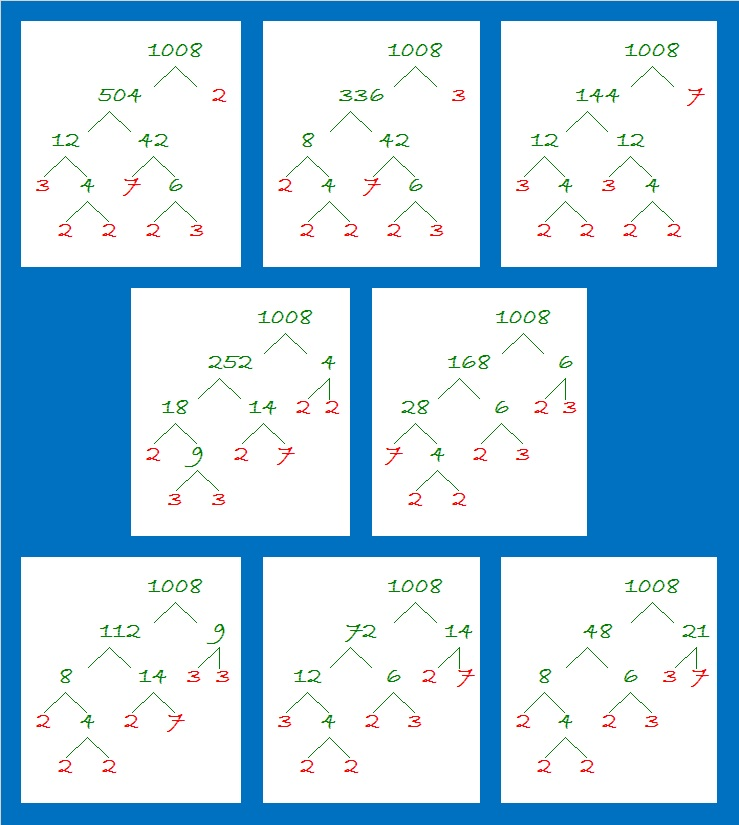Here are some other facts about the number 1008:

1008 is the sum of ten consecutive prime numbers:
79 + 83 + 89 + 97 + 101 + 103 + 107 + 109 + 113 + 127 = 1008

1008 looks interesting when written in some other bases:
It’s 33300 in BASE 4 because 3(4⁴) + 3(4³) + 3(4²) = 3(256 + 64 + 16) = 3(336) = 1008,
4400 in BASE 6 because 4(6³) + 4(6²) = 4(216 + 36) = 4(252) = 1008,
700 in BASE 12 because 7(12²) = 7(144) = 1008
SS in BASE 35 (S is 28 base 10) because 28(35) + 28(1) = 28(36) = 1008
S0 in BASE 36 because 28(36) = 1008

• 1008 is a composite number.
• Prime factorization: 1008 = 2 × 2 × 2 × 2 × 3 × 3 × 7, which can be written 1008 = 2⁴ × 3² × 7
• The exponents in the prime factorization are 4, 2 and 1. Adding one to each and multiplying we get (4 + 1)(2 + 1)(1 + 1) = 5 × 3 × 2 = 30. Therefore 1008 has exactly 30 factors.
• Factors of 1008: 1, 2, 3, 4, 6, 7, 8, 9, 12, 14, 16, 18, 21, 24, 28, 36, 42, 48, 56, 63, 72, 84, 112, 126, 144, 168, 252, 336, 504, 1008
• Factor pairs: 1008 = 1 × 1008, 2 × 504, 3 × 336, 4 × 252, 6 × 168, 7 × 144, 8 × 126, 9 × 112, 12 × 84, 14 × 72, 16 × 63, 18 × 56, 21 × 48, 24 × 42, or 28 × 36
• Taking the factor pair with the largest square number factor, we get √1008 = (√144)(√7) = 12√7 ≈ 31.74902# 960 Factor Trees

### Factor Trees for 960:

960 is the smallest number to have exactly 28 factors. 960 is 2⁶·3·5, so any factor tree made for it will have 6 + 1 + 1 = 8 prime factors. Since 8 is a power of 2, this number, 960, has some beautiful and well-balanced factor trees as well as some that aren’t as good-looking. Here are five of the MANY possible factor trees for 960:### Factors of 960:

• 960 is a composite number.
• Prime factorization: 960 = 2 × 2 × 2 × 2 × 2 × 2 × 3 × 5, which can be written 960 = 2⁶ × 3 × 5
• The exponents in the prime factorization are 6, 1 and 1. Adding one to each and multiplying we get (6 + 1)(1 + 1)(1 + 1) = 7 × 2 × 2 = 28. Therefore 960 has exactly 28 factors.
• Factors of 960: 1, 2, 3, 4, 5, 6, 8, 10, 12, 15, 16, 20, 24, 30, 32, 40, 48, 60, 64, 80, 96, 120, 160, 192, 240, 320, 480, 960
• Factor pairs: 960 = 1 × 960, 2 × 480, 3 × 320, 4 × 240, 5 × 192, 6 × 160, 8 × 120, 10 × 96, 12 × 80, 15 × 64, 16 × 60, 20 × 48, 24 × 40, or 30 × 32
• Taking the factor pair with the largest square number factor, we get √960 = (√64)(√15) = 8√15 ≈ 30.9838668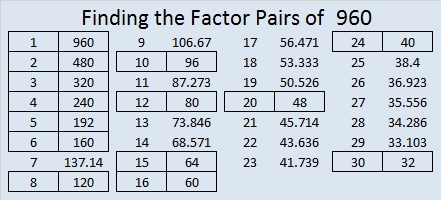### Sum-Difference Puzzles:

60 has six factor pairs. One of those pairs adds up to 17, and  another one subtracts to 17. Put the factors in the appropriate boxes in the first puzzle.

960 has fourteen factor pairs. One of the factor pairs adds up to ­68, and a different one subtracts to 68. If you can identify those factor pairs, then you can solve the second puzzle!The second puzzle is really just the first puzzle in disguise. Why would I say that?

### More about the Number 960:

960 can be written as the difference of 2 squares TEN different ways:

1. 241² – 239² = (241 + 239)(241 – 239) = 480 × 2 = 960
2. 122² – 118² = (122 + 118)(122 – 118) = 240 × 4 = 960
3. 83² – 77² = (83 + 77)(83 – 77) = 160 × 6 = 960
4. 64² – 56² = (64 + 56)(64 – 56) = 120 × 8 = 960
5. 53² – 43² = (53 + 43)(53 – 43) = 96 × 10 = 960
6. 46² – 34² = (46 + 34)(46 – 34) = 80 × 12 = 960
7. 38² – 22² = (38 + 22)(38 – 22) = 60 × 16 = 960
8. 34² – 14² = (34 + 14)(34 – 14) = 48 × 20 = 960
9. 32² – 8² = (32 + 8)(32 – 8) = 40 × 24 = 960
10. 31² – 1² = (31 + 1)(31 – 1) = 32 × 30 = 960

960 is the sum of the sixteen prime numbers from 29 to 97.

It is also the sum of six consecutive prime numbers:
149 + 151 + 157 + 163 + 167 + 173 = 960

960 is the hypotenuse of a Pythagorean triple:
576-768-960 which is (3-4-5) times 192

I like how 960 looks in these other bases:
33000 in BASE 4 because 3(4⁴) + 3(4³) = 3(256 + 64) = 3 × 320 = 960
440 in BASE 15 because 4(15²) + 4(15) = 4(225 + 15) = 4 × 240 = 960
UU in BASE 31 (U is 30 base 10), because 30(31) + 30(1) = 30(31 + 1) = 30 × 32 = 960
U0 in BASE 32 because 30(32) + 0 = 960

OEIS.org informs us that 9 + 6 + 09³ + 6³ + 0³ = 960

# Applying Divisibility Rules to 924

### Divisibility Rules and 924:

Let’s apply some basic divisibility rules to find some of the factors of 924:

1. Like every other counting number, 924 is divisible by 1.
2. Since 924 is even, it is divisible by 2.
3. 9 + 2 + 4 = 15, a number divisible by 3, so 924 is divisible by 3.
4. Its last two digits, 24, is divisible by 4, so 924 is divisible by 4.
5. Its last digit isn’t 0 or 5, so 924 is NOT divisible by 5.
6. 924 is even and divisible by 3, so it is also divisible by 6.
7. Since 92-2(4) = 84, a number divisible by 7, we know that 924 is also divisible by 7.
8. Since its last two digits are divisible by 8, and the third to the last digit, 9, is odd, 924 is NOT divisible 8.
9. 9 + 2 + 4 = 15, a number not divisible by 9, so 924 is NOT divisible by 9.
10. The last digit is not 0, so 924 is NOT divisible by 10.
11. 9 – 2 + 4 = 11, so 924 is divisible by 11.

Thus, 1, 2, 3, 4, 6, 7, and 11 are all factors of 924.

### Factor Cake for 924:

You can see its prime factors easily on the outside of its festive prime factor cake:### Factors of 924:

• 924 is a composite number.
• Prime factorization: 924 = 2 x 2 x 3 x 7 x 11, which can be written 924 = 2² x 3 x 7 x 11
• The exponents in the prime factorization are 2, 1, 1, and 1. Adding one to each and multiplying we get (2 + 1)(1 + 1)(1 + 1)(1 + 1) = 3 x 2 x 2 x 2 = 24. Therefore 924 has exactly 24 factors.
• Factors of 924: 1, 2, 3, 4, 6, 7, 11, 12, 14, 21, 22, 28, 33, 42, 44, 66, 77, 84, 132, 154, 231, 308, 462, 924
• Factor pairs: 924 = 1 x 924, 2 x 462, 3 x 308, 4 x 231, 6 x 154, 7 x 132, 11 x 84, 12 x 77, 14 x 66, 21 x 44, 22 x 42, or 28 x 33,
• Taking the factor pair with the largest square number factor, we get √924 = (√4)(√231) = 2√231 ≈ 30.3973683.### Sum Difference Puzzle:

924 has twelve factor pairs. One of the factor pairs adds up to 65, and a different one subtracts to 65. If you can identify those factor pairs, then you can solve this puzzle!### More about the Number 924:

You may have seen one of its many possible factor trees contained in the first frame of this factor tree for 852,852 from my previous post:924 is in the very center of the 12th row of Pascal’s triangle because 12!/(6!6!) = 924.924 is the sum of consecutive prime numbers: 461 + 463 = 924

I like 924 written in some other bases:
770 BASE 11
336 BASE 17
220 BASE 21
SS BASE 32, S is 28
S0 BASE 33

924 has several sets of consecutive factors. Besides being divisible by the 1st, 2nd, and 3rd triangular numbers (1, 3, and 6), those consecutive factors mean the following:

• 924 is divisible by the 6th triangular number, 21, which is 6(7)/2.
• 924 is divisible by the 11th triangular number, 66, which is 11(12)/2.
• 924 is divisible by the 21st triangular number, 231, which is 21(22)/2.

# 660 My Two-Year Blogiversary

Today is my 2 year blogiversary and my 660th post. Thank you to all my readers and followers. I really appreciate all of you.

What better way is there to celebrate than with a gorgeous cake? Enjoy!

The cake method of finding prime factors makes a beautiful cake for the number 660 because 660 has several prime factors and the largest one, 11, looks like a couple of candles to top it off perfectly.660 has a lot of factors, 24 in fact. 660 is a special number for several reasons:

No number less than 660 has more factors than it does, but 360, 420, 480, 504, 540, 600, and 630 each have just as many.

660 has so many factors that it seems natural for it to between twin primes, 659 and 661.

660 is the hypotenuse of the Pythagorean triple 396-528-660. What is the greatest common factor of those three numbers?

660 is the sum of consecutive prime numbers three different ways. Prime number 101 is in two of those ways:

• 157 + 163 + 167 + 173 = 660
• 101 + 103 + 107 + 109 + 113 + 127 = 660
• 67 + 71 + 73 + 79 + 83 + 89 + 97 + 101 = 660

—————————————————————————————————

• 660 is a composite number.
• Prime factorization: 660 = 2 x 2 x 3 x 5 x 11, which can be written 660 = 2² x 3 x 5 x 11
• The exponents in the prime factorization are 2, 1, 1, and 1. Adding one to each and multiplying we get (2 + 1)(1 + 1)(1 + 1)(1 + 1) = 3 x 2 x 2 x 2 = 24. Therefore 660 has exactly 24 factors.
• Factors of 660: 1, 2, 3, 4, 5, 6, 10, 11, 12, 15, 20, 22, 30, 33, 44, 55, 60, 66, 110, 132, 165, 220, 330, 660
• Factor pairs: 660 = 1 x 660, 2 x 330, 3 x 220, 4 x 165, 5 x 132, 6 x 110, 10 x 66, 11 x 60, 12 x 55, 15 x 44, 20 x 33, or 22 x 30
• Taking the factor pair with the largest square number factor, we get √660 = (√4)(√165) = 2√165 ≈ 25.690465.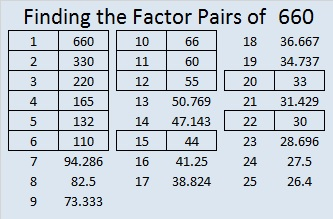—————————————————————————————————

# 420 Factor Trees

Did you know that the sum of all the prime numbers between 100 and 110 equals 420? Yes, 101 + 103 + 107 + 109 = 420.

Since 20 × 21 = 420, we know that 420 is the sum of the first 20 EVEN numbers. Thus,

• 2 + 4 + 6 + 8 + 10 + 12 + 14 + 16 + 18 + 20 + 22 + 24 + 26 + 28 + 30 + 32 + 34 + 36 + 38 + 40 = 420.

420 is the smallest number that can be divided evenly by all the natural numbers from 1 to 7.

420 has a LOT of factors, more than most people would think it does.  In fact, of all of the numbers from 1 to 420, there is only one number, 360, that has as many factors as 420 has.

There are 4 different prime numbers that can divide evenly into 420. Here are those factor trees:Many, but not all, of the factors of 420 are listed somewhere on those four trees.

• 420 is a composite number.
• Prime factorization: 420 = 2 x 2 x 3 x 5 x 7, which can be written 420 = (2^2) x 3 x 5 x 7
• The exponents in the prime factorization are 2, 1, 1, and 1. Adding one to each and multiplying we get (2 + 1)(1 + 1)(1 + 1)(1 + 1) = 3 x 2 x 2 x 2 = 24. Therefore 420 has exactly 24 factors.
• Factors of 420: 1, 2, 3, 4, 5, 6, 7, 10, 12, 14, 15, 20, 21, 28, 30, 35, 42, 60, 70, 84, 105, 140, 210, 420
• Factor pairs: 420 = 1 x 420, 2 x 210, 3 x 140, 4 x 105, 5 x 84, 6 x 70, 7 x 60, 10 x 42, 12 x 35, 14 x 30, 15 x 28, or 20 x 21
• Taking the factor pair with the largest square number factor, we get √420 = (√4)(√105) = 2√105 ≈ 20.4939Each factor pair, except 1 x 420, can make its own factor tree. Here are some factor trees featuring the other seven factor pairs:If I hadn’t made all the prime numbers in red, it’s possible that one or more of the prime numbers might get forgotten. That is why I prefer the cake method for finding the prime factorization of a number. All of the prime numbers are listed in numerical order on the outside of the cake.